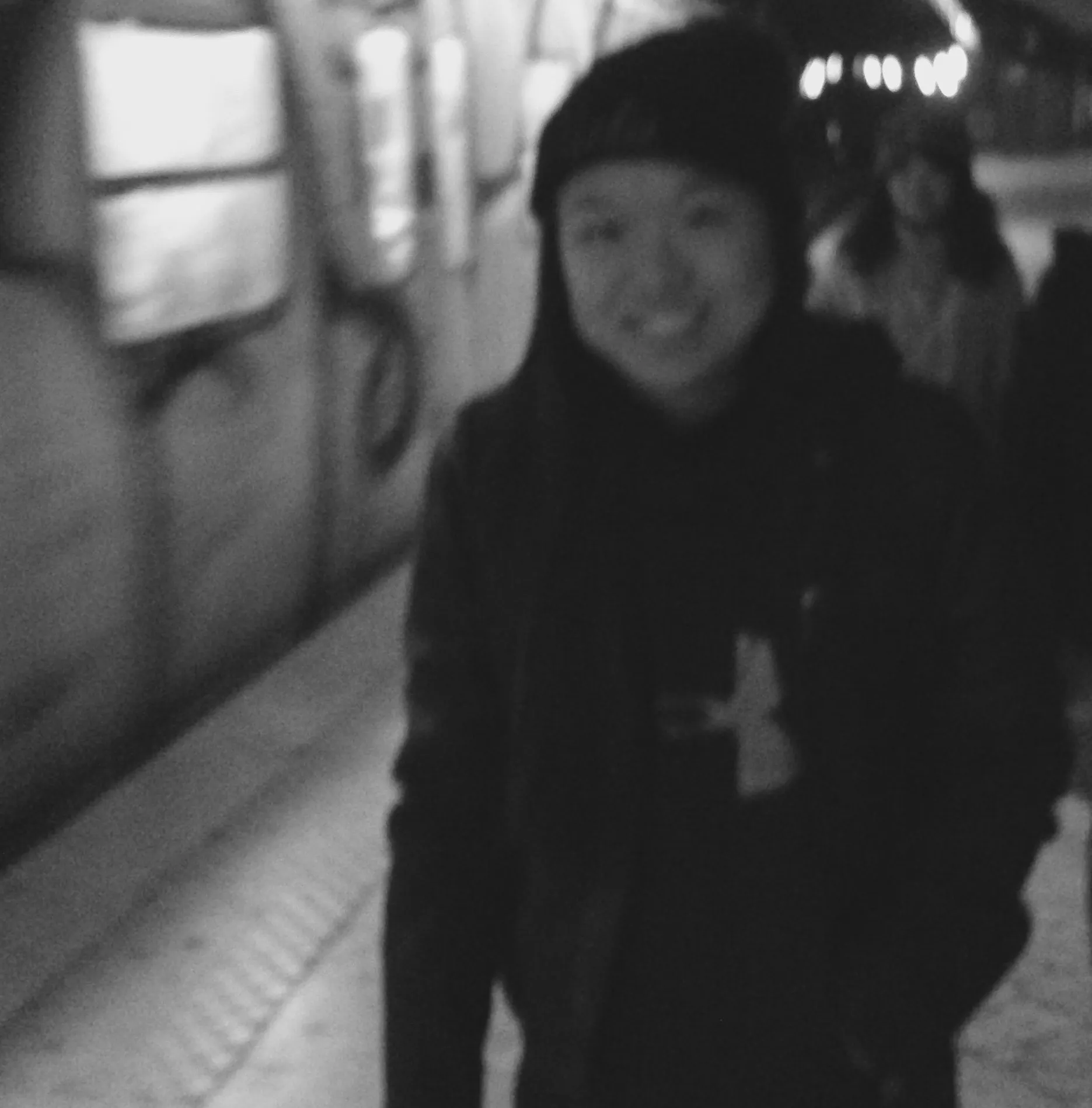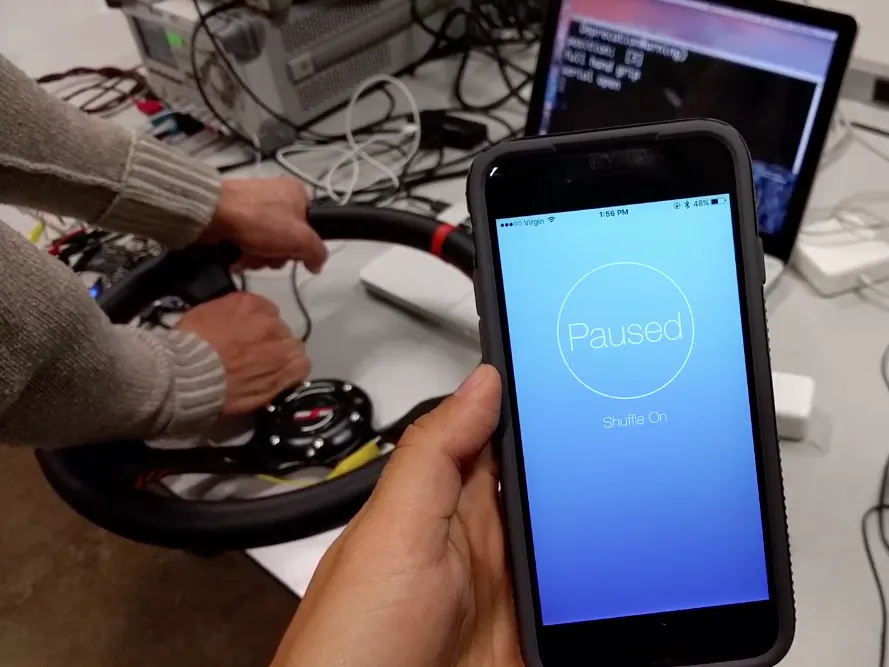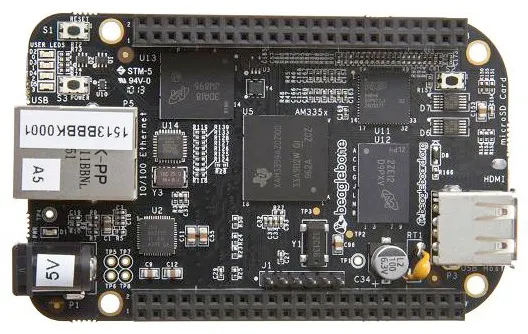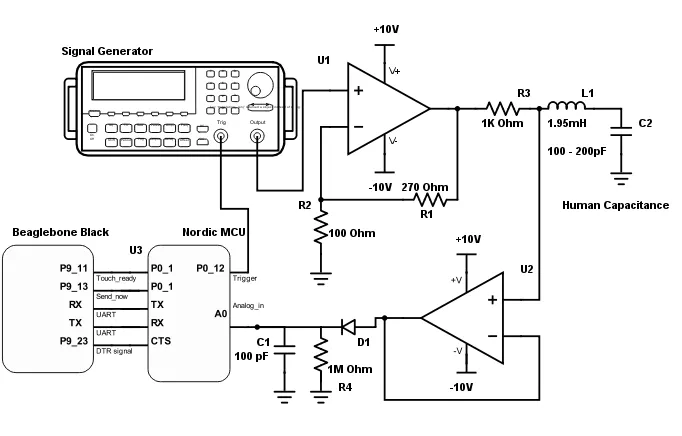Published

# CapTouch Steering Wheel Touch Interface

Add a touch interface to the steering wheel of any car to control music on your phone!## Things used in this project

### Hardware components

 Nordic nRF51-DK mbed board
×1BeagleBoard.org BeagleBone Black
×1
×1
 LT1360 Op-Amp
×2

## Schematics

### System Diagram## Code

### Classifier run on Beaglebone

Python
```import warnings
warnings.filterwarnings("ignore", category=RuntimeWarning)
import numpy as np
import matplotlib.pyplot as plt
from sklearn import svm
import serial
import time

#setup UART connection
print "setup"
UART.setup("UART1")
ser1 = serial.Serial(port = "/dev/ttyO1", baudrate=9600)

dtr = "P9_23"
send_again = "P9_22"
result0 = "P9_13"
result1 = "P9_11"

#setup
GPIO.setup(dtr, GPIO.OUT)
GPIO.setup(send_again, GPIO.OUT)
GPIO.setup(result0, GPIO.OUT)
GPIO.setup(result1, GPIO.OUT)
#initialize
GPIO.output(send_again, GPIO.LOW)
GPIO.output(result0, GPIO.LOW)
GPIO.output(result1, GPIO.LOW)

data = []
clf = svm.SVC(kernel = 'linear', C=1.0)

def isFloat(string):
try:
float(string)
return True
except ValueError:
return False

def train_data():
global clf

#read in and parse our CSVs for training data
train_data_notouch = open("notouch.csv", 'r')				#0
train_data_onefinger = open("onehand_onetouch.csv", 'r')  				#1
train_data_twotouch = open("onehand_twotouch.csv", 'r') 				#2
train_data_fullgrip = open("onehand_fullgrip.csv", 'r') 				#3
train_data_twohands = open("twohands.csv", 'r') 				#4

train_data_notouch = list(train_data_notouch)
train_data_onefinger = list(train_data_onefinger)
train_data_twotouch = list(train_data_twotouch)
train_data_fullgrip = list(train_data_fullgrip)
train_data_twohands = list(train_data_twohands)

for i,line in enumerate(train_data_notouch):
train_data_notouch[i] = train_data_notouch[i].rstrip('\n').split(',')
train_data_notouch[i] = train_data_notouch[i][0:70]

for i,line in enumerate(train_data_onefinger):
train_data_onefinger[i] = train_data_onefinger[i].rstrip('\n').split(',')
train_data_onefinger[i] = train_data_onefinger[i][0:70]

for i,line in enumerate(train_data_twotouch):
train_data_twotouch[i] = train_data_twotouch[i].rstrip('\n').split(',')
train_data_twotouch[i] = train_data_twotouch[i][0:70]

for i,line in enumerate(train_data_fullgrip):
train_data_fullgrip[i] = train_data_fullgrip[i].rstrip('\n').split(',')
train_data_fullgrip[i] = train_data_fullgrip[i][0:70]

for i,line in enumerate(train_data_twohands):
train_data_twohands[i] = train_data_twohands[i].rstrip('\n').split(',')
train_data_twohands[i] = train_data_twohands[i][0:70]

#np_train_data_notouch = np.array(train_data_notouch)
#np_train_data_notouch = np_train_data_notouch.astype(float)
#mins_water = np.min(np_train_data_notouch)
#print mins_water

training_input = []
training_output = []
#train our classifier
for k in range(1,4):
for i in range(0,3):
training_input.append(train_data_notouch[i])
training_output.append(0)
for i in range(0,3):
training_input.append(train_data_onefinger[i])
training_output.append(1)
for i in range(0,3):
training_input.append(train_data_twotouch[i])
training_output.append(2)
for i in range(0,3):
training_input.append(train_data_fullgrip[i])
training_output.append(3)
for i in range(0,3):
training_input.append(train_data_twohands[i])
training_output.append(4)

#fit the data
X = training_input
y = training_output
clf.fit(X,y)
print "training score:", clf.score(X,y)

ser1.close()
ser1.open()

data_array = [];

if ser1.isOpen():
print 'serial open'
count = 0

GPIO.output(dtr, GPIO.HIGH)
GPIO.output(dtr, GPIO.LOW)
GPIO.output(send_again, GPIO.HIGH) #tell mbed to send

count = 0
if data_array!="start":
data_array = []
continue
else:
data_array.pop(0);
data = data_array
data_array = []
break

else:
count = count+1

#ser1.close()				#should we keep serial opened or closed?

def run_classifier():
data_float = map(float, data)
#print "floats to run classifier on: ", data		#print the data if it is new
result = clf.predict(data_float)
print "position: ", result

if result==0:
print "no hands"
elif result==1:
print "one finger touch"
elif result==2:
print "two finger touch"
elif result==3:
print "full hand grip"
elif result==4:
print "two hands on steering wheel"
else:
print "n/a"

GPIO.output(touch_ready, GPIO.HIGH)	#tell mbed interrupt that classification is done
"""
if data_float > 0.5:
result = 2
if data_float <= 0.5:
result = 1
"""
ser1.write(chr(result))

if __name__ == '__main__':

train_data()
time.sleep(1)

while(1):
run_classifier()
GPIO.output(send_again, GPIO.LOW)
"""
while 1:
print "high"
print "low"
time.sleep(3)
"""

```

### Data collection and BLE interface

C/C++
```#include "mbed.h"
#include "ble/BLE.h"
#include "CaptouchService.h"
#include "Serial.h"
#include "BufferedSerial.h"

#define BTON 1
//#define BTEST 2

InterruptIn button1(BUTTON1);
InterruptIn button2(BUTTON2);
InterruptIn button3(BUTTON3);
InterruptIn button4(BUTTON4);

DigitalOut led1(LED1);
DigitalOut led2(LED2);
DigitalOut led3(LED3);
DigitalOut led4(LED4);

DigitalOut trigger(P0_12);
DigitalOut test_out(P0_13);
InterruptIn send_now_interrupt(P0_15);

Timer t;
static uint8_t touchState = 0;

#if defined(BTON)
const static char     DEVICE_NAME[] = "captouch";
static const uint16_t uuid16_list[] = {CaptouchService::CAPTOUCH_SERVICE_UUID};
static CaptouchService *captouchServicePtr;
#endif

char end_buffer = "end";
char buffer;
char start_buffer = "start";
int send_now = 0;
int eventIncoming = 0;

Serial pc(USBTX, USBRX);
BufferedSerial beaglebone(P0_9, P0_11);
char touchStateChar = 'a';

#if defined(BTEST)
void button1RiseCallback(void)
{
led1 = !led1;
test_out = 0;
}

void button2RiseCallback(void)
{
led2 = !led2;
test_out = 1;
}

void button3RiseCallback(void)
{
led3 = !led3;
test_out = 0;
}

#else
void button1RiseCallback(void)
{
test_out = 0;
}

void button2RiseCallback(void)
{
led2 = !led2;
test_out = 1;
}

void button3RiseCallback(void)
{
led1 = !led1;
test_out = 0;
}
#endif

#if defined (BTON)
void disconnectionCallback(const Gap::DisconnectionCallbackParams_t *params)
{
}

void onBleInitError(BLE &ble, ble_error_t error)
{
printf("Error initializing BLE\n\r");
}

void bleInitComplete(BLE::InitializationCompleteCallbackContext *params)
{
BLE&        ble   = params->ble;
ble_error_t error = params->error;

if (error != BLE_ERROR_NONE) {
/* In case of error, forward the error handling to onBleInitError */
onBleInitError(ble, error);
return;
}

/* Ensure that it is the default instance of BLE */
if(ble.getInstanceID() != BLE::DEFAULT_INSTANCE) {
return;
}

ble.gap().onDisconnection(disconnectionCallback);

/* Setup primary service */
captouchServicePtr = new CaptouchService(ble, 0x00);

}
#endif

}

void sendNow() {
send_now = 1;
}

int main(void)
{
uint8_t old_touchState = 0x00;
beaglebone.baud(9600);
float data_float, elapsed, start_time, end_time;
int i;
led1 = 1;
led2 = 1;
led3 = 1;
led4 = 1;
test_out = 1;

#if defined (BTON)
BLE &ble = BLE::Instance();
ble.init(bleInitComplete);
#endif

t.start();

#if defined (BTON)
while (ble.hasInitialized()  == false) { }
#endif

send_now_interrupt.rise(&sendNow);
button1.rise(&button1RiseCallback);
button2.rise(&button2RiseCallback);
button3.rise(&button3RiseCallback);

while(1) {
i = 0;
//t.start();
if (send_now==1) {
//__disable_irq();
while (i < 70) {
if (i==0) {
beaglebone.puts(start_buffer);
}
if (i==10) {
trigger = 1;                //give a couple samples leeway before pulsing trigger
}
sprintf(buffer, "%f", data_float);
beaglebone.puts(buffer);
memset(buffer, 0, sizeof(buffer));
i++;
trigger = 0;
}

//beaglebone.puts(timebuf);
beaglebone.puts(end_buffer);
//__enable_irq();
send_now = 0;
//sprintf(timebuf, "%f", end_time - start_time);
//printf("%f", end_time - start_time);
}
{
touchStateChar = beaglebone.getc();
}

#if defined (BTON)
if (ble.getGapState().connected) {
touchState = int(touchStateChar) - 256; //convert to uint8
if (old_touchState != touchState) {
captouchServicePtr->updateTouchState(touchState);
old_touchState = touchState;
led4 = !led4;
}
}
#endif
}
led3 = !led3;
//wait(0.3);

}

}
```

## Credits

### Priscilla Cheng

0 projects • 3 followers